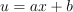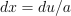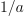/NumPy 1.17

# numpy.polynomial.hermite_e.hermeint

`numpy.polynomial.hermite_e.hermeint(c, m=1, k=[], lbnd=0, scl=1, axis=0)` [source]

Integrate a Hermite_e series.

Returns the Hermite_e series coefficients `c` integrated `m` times from `lbnd` along `axis`. At each iteration the resulting series is multiplied by `scl` and an integration constant, `k`, is added. The scaling factor is for use in a linear change of variable. (“Buyer beware”: note that, depending on what one is doing, one may want `scl` to be the reciprocal of what one might expect; for more information, see the Notes section below.) The argument `c` is an array of coefficients from low to high degree along each axis, e.g., [1,2,3] represents the series `H_0 + 2*H_1 + 3*H_2` while [[1,2],[1,2]] represents ```1*H_0(x)*H_0(y) + 1*H_1(x)*H_0(y) + 2*H_0(x)*H_1(y) + 2*H_1(x)*H_1(y)``` if axis=0 is `x` and axis=1 is `y`.

Parameters: `c : array_like` Array of Hermite_e series coefficients. If c is multidimensional the different axis correspond to different variables with the degree in each axis given by the corresponding index. `m : int, optional` Order of integration, must be positive. (Default: 1) `k : {[], list, scalar}, optional` Integration constant(s). The value of the first integral at `lbnd` is the first value in the list, the value of the second integral at `lbnd` is the second value, etc. If `k == []` (the default), all constants are set to zero. If `m == 1`, a single scalar can be given instead of a list. `lbnd : scalar, optional` The lower bound of the integral. (Default: 0) `scl : scalar, optional` Following each integration the result is multiplied by `scl` before the integration constant is added. (Default: 1) `axis : int, optional` Axis over which the integral is taken. (Default: 0). New in version 1.7.0. `S : ndarray` Hermite_e series coefficients of the integral. ValueError If `m < 0`, `len(k) > m`, `np.ndim(lbnd) != 0`, or `np.ndim(scl) != 0`.

#### Notes

Note that the result of each integration is multiplied by `scl`. Why is this important to note? Say one is making a linear change of variablein an integral relative to `x`. Then, so one will need to set `scl` equal to- perhaps not what one would have first thought.

Also note that, in general, the result of integrating a C-series needs to be “reprojected” onto the C-series basis set. Thus, typically, the result of this function is “unintuitive,” albeit correct; see Examples section below.

#### Examples

```>>> from numpy.polynomial.hermite_e import hermeint
>>> hermeint([1, 2, 3]) # integrate once, value 0 at 0.
array([1., 1., 1., 1.])
>>> hermeint([1, 2, 3], m=2) # integrate twice, value & deriv 0 at 0
array([-0.25      ,  1.        ,  0.5       ,  0.33333333,  0.25      ]) # may vary
>>> hermeint([1, 2, 3], k=1) # integrate once, value 1 at 0.
array([2., 1., 1., 1.])
>>> hermeint([1, 2, 3], lbnd=-1) # integrate once, value 0 at -1
array([-1.,  1.,  1.,  1.])
>>> hermeint([1, 2, 3], m=2, k=[1, 2], lbnd=-1)
array([ 1.83333333,  0.        ,  0.5       ,  0.33333333,  0.25      ]) # may vary
```

© 2005–2019 NumPy Developers
Licensed under the 3-clause BSD License.
https://docs.scipy.org/doc/numpy-1.17.0/reference/generated/numpy.polynomial.hermite_e.hermeint.html